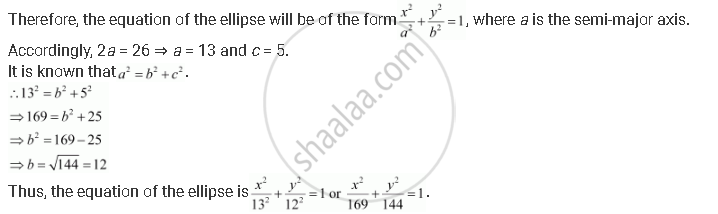CBSE (Arts) Class 11CBSE
Share
Notifications

View all notifications
Books Shortlist
Your shortlist is empty

# Find the Equation for the Ellipse that Satisfies the Given Conditions: Length of Major Axis 26, Foci (±5, 0) - CBSE (Arts) Class 11 - Mathematics

Login
Create free account

Forgot password?
ConceptEllipse Standard Equations of an Ellipse

#### Question

Find the equation for the ellipse that satisfies the given conditions: Length of major axis 26, foci (±5, 0)

#### Solution

Length of major axis = 26; foci = (±5, 0).

Since the foci are on the x-axis, the major axis is along the x-axis.Is there an error in this question or solution?

#### APPEARS IN

Solution Find the Equation for the Ellipse that Satisfies the Given Conditions: Length of Major Axis 26, Foci (±5, 0) Concept: Ellipse - Standard Equations of an Ellipse.
S